[BACK]
 Intelligent Automation & Soft ComputingDOI:10.32604/iasc.2022.021581Article

Coronavirus Decision-Making Based on a Locally SMα -Generalized Closed Set

1Department of Mathematics and Statistics, College of Science, Taif University, Taif, 21944, Saudi Arabia
2Department of Mathematics, College of Science, University of Jeddah, Jeddah, Saudi Arabia
3Department of Mathematics, College of Science, King Khalid University, Abha, Saudi Arabia
4Department of Mathematics, College of Science and Arts, Najran University, Najran, 66445, Saudi Arabia
*Corresponding Author: M. A. El Safty. Email: m.elsafty@tu.edu.sa
Received: 06 July 2021; Accepted: 07 August 2021

Abstract: Real-world applications now deal with a massive amount of data, and information about the world is inaccurate, incomplete, or uncertain. Therefore, we present in our paper a proposed model for solving problems. This model is based on the class of locally generalized closed sets, namely, locally simply* alpha generalized closed* sets and locally simply* alpha generalized closed** sets (briefly, LSMαGC -sets and LSMαGC -sets), based on simply* alpha open set. We also introduce various concepts of their properties and their relationship with other types, and we are studying several of their properties. Finally, we apply the concept of the simply* alpha open set to illustrate the importance of our method in decision-making for information systems about the infections of Coronavirus in humans. In fact, we were able to decide the impact factors of Coronavirus infection. The results were also programmed using the MATLAB program. Therefore, it is recommended that our proposed concept be used in future decision-making.

Keywords: Corona virus; simply* alpha open sets; locally simply* alpha generalized closed*sets; decision making

1  Introduction

The most recent Coronavirus epidemic was an outbreak in China at the start of 2019. China increased its national public-health response to the highest level on January 23, 2020. A variety of public social distancing initiatives to minimize the transmission rate of Corona virus have been introduced as part of the emergency response . As a result, many papers were published by several researchers to study and analyze this virus, such as .

In diverse research areas, the mathematical modeling of vagueness and ambiguity has become an increasingly important topic. There exists another statistical instrument that has been utilized in many aspects of life [8,9]. Topology is an important branch of mathematics which contains several subfields such as soft topology, algebraic topology, and differential topology [10,11]. These subfields increase the limit of the topology applications . The field of topology has many results and concepts, which can help us to discover the hidden information of data, composed of real-life applications . The topological methods are thus compatible with the processing static methods and geometric representation the concept of a locally closed set-in topological space, introduced by Balachandran et al. . Ganster et al.  continued to study of locally closed sets.

Our aim in this paper is to introduce three new classes of sets called LSMαGC -closed-sets, LSMαGC, and LSMαGC -sets which are contained in GLC - sets by using the notions of SMα -open sets and SMα -closed sets which is new classes. We shall define these classes in this paper. Also, we introduce some different classes of SMαG -closed sets and SMαG -open sets and study some of their characteristics. This method is used to identify and take the optimal decision for any life problem using near open sets named the class of simply stare alpha Star open sets, which is a new method that is considered as an essential tool for processing information for any life problem and is useful for any decision maker to take the appropriate decision for it. For example, the current application can help in making the right decision for patients, the algorithm used in the manuscript can be applied to any number of patients or objects.

Finally, we explain the importance of the proposed method in the medical sciences for application in decision making problems. In fact, a medical application has been introduced in the decision making process of COVID-19 Medical Diagnostic Information System with the algorithm. This application may help the world to reduce the spread of Coronavirus.

The paper is structured as follows: The basic concepts of the locally generalized closed set and locally simply* α -generalized closed sets are introduced in Section Two and Three. The implementation of COVID-19 for each subclass of attributes in the information systems and comparative analysis is presented in Section Four. Section Five concludes and highlights future scope.

2  Preliminaries

In this section, the present study is inspired by pointing out locally generalized closed set blind spots.

Definition 2.1  A subset A^ of a space W^ is called g-closed if cL(A^)  G wherever A^  G, and G is open

Definition 2.2 A subset A^ of a space (W^,T) it’s called,

i.   Locally-closed  if A^=G  ϝ where G  T and ϝ is closed-set in (W^,T)

ii.   Generalized locally closed  (briefly GLC -set) if A^=G  ϝ whereas G  GO(W^) and ϝ GC(W) .

iii.    GLC -closed  if there is a g-open set G and closed ϝ of (W^,T) whereas A^=G  ϝ .

iv.    GLC -set  if there is an open-set G and a g-closed set ϝGCO(W^) whereas A^=G  ϝ The collection of all locally GLC -sets (resp. GLC -sets, GLC -sets, SMαG -sets, closed sets, and g-closed sets) will denoted by GLC(W^) (resp. GLC(W^),GLC(W^) , SMαGC(W^),C(W^),GC(W^) .

Definition 2.3  A topological space (W^,T) is called g-submaximal if every dense is g-open.

3  Locally Simply*α-Generalized Closed Sets

In this section, we shall introduce a new class of generalization say LSMαGC -sets and we study some properties of this class.

Definition 3.1 A subset A^ of a space (W^,T) it’s called SMα -open set if A^  {W^,ϕ,G  N where G is proper α-open set and N is nowhere dense } and the complement of the class of SαM open set is called SαM closed set and well denoted by SMαO(W^) and its complement is denoted by SMαC(W^) .

Definition 3.2 A subset A^ of a topological space (W^,T) is called:

i.   Simply alpha interior (shortly, SMαint(A^) ) is the largest simply* alpha open set contained in A^ and A^ such that G  A^} .

ii.   Simply alpha closure (briefly, SMαcL(A^) ) is the smallest simply* alpha closed-set contained in A^ and A^ such that A^  ϝ} .

Definition 3.3 A subset A^ of a topological space (W^,T) is called simply* alpha dense (briefly, SMα -dense) if SMαcL(A^)=W^ .

Remark 3.1 The following Fig. 1 shows the relation between a new class of generalization and the other types of generalization of sets such that the implication in this diagram cannot be reversible as indicated in Example 3.1.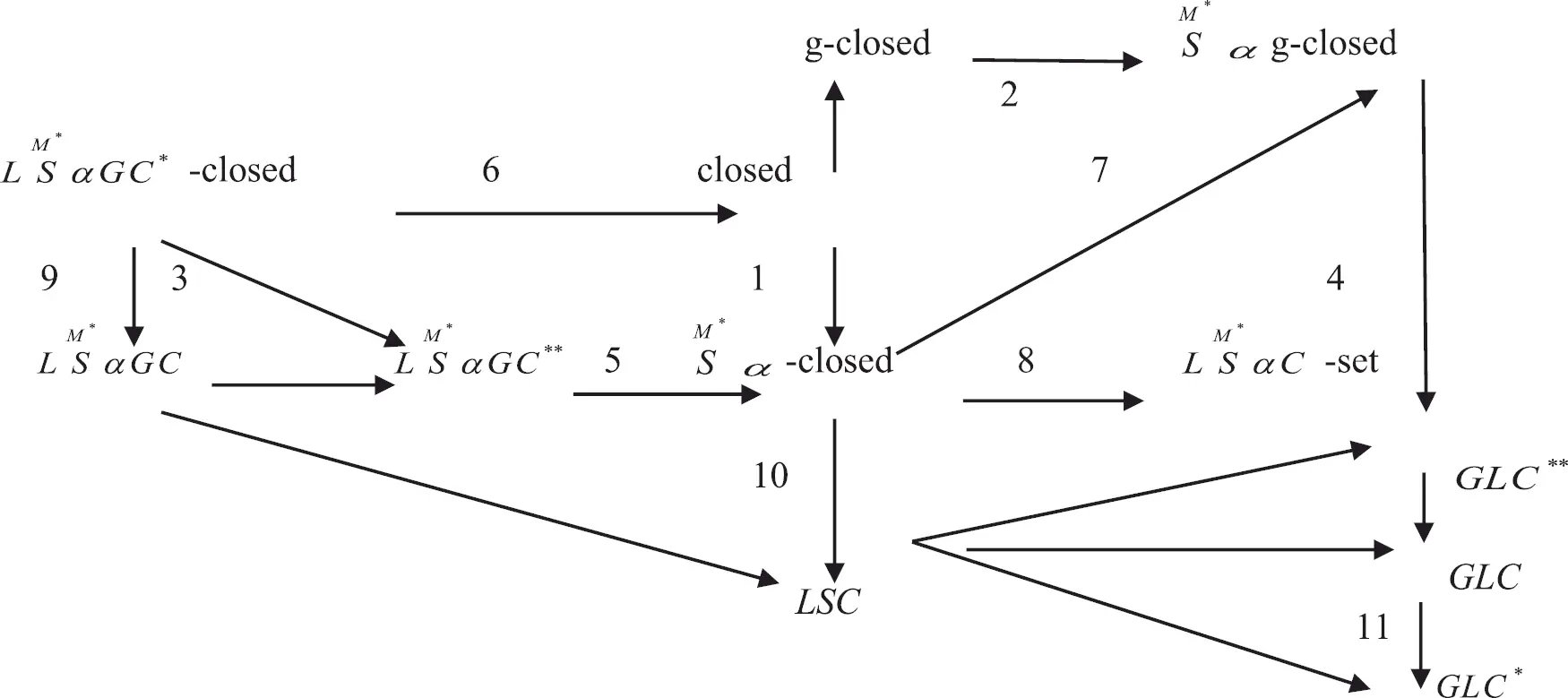Figure 1: Shows the relation between a new class of generalized closed-sets

Definition 3.4 A subset A^ of a space (W^,T) it’s called.

i.   Simply α -generalized-closed (shortly, SMαG -closed) set if SMαcL(A^)  G,A^  G where G is open-set.

ii.   Simply α -generalized-open (shortly, SMαG -open) set if ϝ SMαint(A^),ϝ  A^ where ϝ is closed set.

iii.   Locally SMα -generalized closed (shortly, LSMαGC ) sets if there is a proper SMαG -open set G and proper SMαG -closed set ϝ of (W^,T) whereas A^=G  ϝ.

iv.   Locally simply α -generalized closed*-sets (shortly, LSMαGC -sets) if there is a proper SMαG -open set G and proper SMα -closed set ϝ of (W^,T) whereas A^=G  ϝ.

v.   Locally simply α -generalized closed**sets (shortly, LSMαGC -sets) if there exists a proper SMα -open-set G and proper SMαG -closed set ϝ of (W^,T) wherever A^=G  ϝ.

vi.   Locally simply*α -closed set (shortly, LSMC -set) if A^=G  ϝ wherever G is SMα -open set and ϝ is SMα -closed sets.

The collection of all locally simply* alpha generalized closed set (resp. locally simply* alpha generalized closed* set, and locally simply* alpha generalized closed** set) of a universe set W^ is denoted by LSMαGC(W^), (resp. LSMαGC(W^),LSMαGC(W^), and LSMC(W^) ) is denoted by LSMαGC(W^) (resp. LSMαGC(W^) , LSMαGC(W^),(W^), and LSMC(W^) ).

Example 3.1 Let W^={a,b,c,d} , T={W^,ϕ,{c},{a,b},{a,b,c}}. Then we have,

(implication 3) A set {a,d}  LSMαGC(W^) but {a,d}  LSMαGC(W^).

(implications 6, 7) A set {b,d}  GLC(W^) but {b,d}  GLC(W^), but {a,b,c}  LSMαGC(W^) .

(implication 5, 1) {a}  LSMαGC(W^) but {a}  SMαC(W^) , and {a,b}  SMαC(W^) but {a,b}  C(W^).

(implication 6, 2, 4) {a,b}  LSMαGC(W^) but {a,b}  C(W^) , {a}  SMαGC(W^) but {a}  GC(W^,T),{a,b,c}  GLC(W^) but {a,b,c}  SMαGC(W^).

(implication 8, 9, 10, 11) A set {a,b,c}  LSMαC(W^), but {a,b,c}  SMαC(W^) , {a,b,c}  LSMαGC(W^) but {a,b,c}  LSMαGC(W^) . Also there exists a set {b}  SMαGC(W^) but it is not {b}  SMαC(W^).

Remark 3.2 For any topological space (W^,T) then we have,

i.    LSMαGC -sets and α g-closed sets are not comparable.

ii.    SMαG -closed sets and LSMαGC -closed sets are not comparable.

iii.   α g-closed sets and LSMαGC -closed sets are not comparable.

iv.    LSMαC closed sets and α g-closed sets are not comparable.

v.   g-closed sets and GLC -closed sets are not comparable.

vi.    SMαG -closed sets and GLC -closed sets are not comparable.

vii.   LSC and LSMαGC -sets are not comparable.

viii.    LSMC and g-closed are not comparable.

ix.    LSMαC set and LSMαGC sets are not comparable.

x.    LSMαC set and LSMαGC sets are not comparable.

Example 3.2 From example 3.1 we have.

i.   There exist a set {c}  LSMαGC(W^) but {c}  GC(W^) and there exists a set {b,c,d}  αGC(W^) but {b,c,d}  LSMαGC(W^) .

ii.   There exists a set {b,c}  LSMαGC(W^,T) but {b,c}  SMαGC(W^,T) , and there exists a set {a,c,d}  SMαGC(W^,T) but {a,c,d}  LSMαGC(W^,T).

iii.   There exist a set {b}  αGC(W^) but {b}  LSMαGC(W^), and there exists a set {b,c,d}  αGC(W^), but {b,c,d}  LSMαGC(W^).

iv.   There exists a set {c}  LSMαC(W^) but {c}  αGC(W^), and there exists a set {b,d}  αGC(W^), but {b,d}  LSMαC(W^).

v.    LSMC{b,d}  αGC(W^) but {b,d}  GLC(W^). and there exists a set {c}  GLC(W^) but {c}  GLC(W^).

vi.   There exists a set {a,c}  GLC(W^), but {a,c}  SMαGC(W^), and there exists a set {b,c,d}  SMαGC(W^), but {b,c,d}  GLC(W^).

vii.   There exists a set {a}  LSMαGC(W^), but {a}  LδC(W^), and there exist a set {a,b,c}  LδC(W^), but {a,b,c}  LSMαGC(W^).

viii.   There exists a set {a,b,c}  LSMαC(W^), but {a,b,c}  GC(W^), and there exists a set {b,d}  GC(W^), but {b,d}  LSMC(W^).

ix.   There exists a set {a,b,c}  LSMαC(W^), but {a,b,c}  LSMαGC(W^), and there exists a set {a}  LSMαGC(W^), but {a}  LSMαC(W^).

x.   There exists a set {a,b,c}  LSMαC(W^), but {a,b,c}  LSMαGC(W^), and there exists a set {a}  LSMαGC(W^), but {a}  LSMαC(W^) .

Definition 3.5 A subset A^ of a space (W^,T) ; it is called SMα -closure of A^ (resp. SMα -interior) briefly as SMαcL(A^) (resp. SMαint(A^) ) is defined by SMαcL(A^)=  {ϝ  A^ , such that ϝ SMC(W^,T)} , and SMαint(A^)={G  A^, such that G  SMαO(W^,T)} .

Theorem 3.1 The collection of all LSMαGC(W^) is not true under finite union; the collection of LSMαGC(W^) is not true under finite union.

The following example indicates this theorem;

i.   From Example 2.1 we get that there exists a set {b},{c}  LSMαGC(W^) but {b}{c}={b,c}  LSMαGC(W^) .

ii.   Let {c},{a,b}  LSMαGC(W^) but {c}{a,b}={a,b,c}  LSMαGC(W^) .

Remark 3.3 From the above result, the classes of LSMαGC(W^) and LSMαGC(W^) are not form supra topology.

Theorem 3.2 For a subset A^ of a space (W^,T) then the following statements are equivalent.

i.    A^  LSMαGC(W^).

ii.    A^  G  SMαcL(A^) for some proper SMα g-open set G .

iii.    SMαcL(A^)A^ is proper SMαG -closed.

iv.    A^(W^SMαcL(A^)) is proper SMα g-open.

Proof : i) → ii) and A^  LSMαGC(W^,T) then A^=G  ϝ where G is proper SMαG -open and ϝ is proper SMα -closed, A^  ϝ implies SMαcL(A^)  ϝ and also

A^=G  ϝ  G  SMαcL(A^) and A^  SMαcL(A^),A^  G implies A^  G  SMαcL(A^) hence A^=G  SMαcL(A^).

ii ) → i ) Since G is proper SMαG -open and SMαcL(A^) is SMαG -closed, G  SMαcL(A^)  LSMαGC(W^,T) by definition 2.1.

ii ) → iii) Since SMαcL(A^)A^=SMαcL(A^)  (W^A^) which is LSMαG -closed but A^=G  SMαcL(A^) then SMαcL(A^)  (W^(G  SMαcL(A^))=SMαcL(A^)  (W^G)(W^SMαcL(A^)) , SMαcL(A^)A^=SMαcL(A^)  (W^G˘)SMαcL(A^)  (W^SMαcL(A^))=SMαcL(A^)  (W^G)ϕ=SMαcL(A^)  (W^G) by lemma 2.1 so SMαcL(A^)A^ is SMαG -closed.

iii ) → ii ) Let U=W^(SMαcL(A^)A^) then U is SMαG -open and hence A^=U  SMαcL(A^).

iii ) → iv) Let ϝ=SMαcL(A^)A^ is proper SMαG -closed then ϝ=SMαcL(A^)  (W^A^) , W^ϝ=(W^SMαcL(A^))  A^ , W^ϝ is proper SMαG -open.

iv ) → iii ) Let U=A^  (W^SMαcL(A^)) then (W^U)=(W^A^)  SMαcL(A^)=SMαcL(A^)A^ is proper SMαG -closed and hence W^U=SMαcL(A^)A^.

Remark 3.4 It is not true that A^  LSMαGC(W^,T)SMint(A^  (W^SMαcL(A^))  A^ in fact shown as the next example 3.3,

Example 3.3 Let W^={a,b,c},T={W^,ϕ,{a},{a,c}} and let A^={b} be subset of (W^,T) then SMint(A^  (W^SMαcL(A^))=SMint({b,c})=ϕ  A^ and A^  LSMαGC(W^,T).

Definition 3.6 A topological space (W^,T) is called SMαG -submaximal if each SMα -dense is SMαG -open.

Proposition 3.1 A topological space (W^,T) is called SMαG -sub-maximal if P(W^)=LSMαGC(W^,T).

Proof. Let A^  P(W^) and U=A^  (W^SMαcL(A^)) then W^=SMαcL(U)

i.e., U is SMα -dense then U is proper SMαG -open by theorem 2.1 U  LSMαGC(W^,T) and hence P(W^)=LSMαGC(W^,T). Conversely, let A^ is SMα -dense subset of (W^,T) then from (4) in theorem 2.1 then A^  (W^SMαcL(A^))=A^ and A^  LSMαGC(W^,T) and hence A^ is proper SMαG -open implies that a space (W^,T) is sub-maximal.

Proposition 3.2 For a subset A^ of (W^,T) if A^  LSMαGC(W^,T) then there exists a proper SMαG -open set G such that A^  G  SMαcL(A^).

Proof. Let A^  LSMαGC(W^,T) then there are both a proper SMαG -open set G and proper SMαG -closed set ϝ such that A^=G  ϝ, as A^  SMαcL(A^),A^  G  G  SMαcL(A^) , G  ϝ G  SMαcL(A^) , A^  G  SMαcL(A^).

4  Application via Simply Alpha Open Set-in Decision Making of Coronavirus

In this section, we provide an application of our approaches for decision making for information systems on Coronavirus infections. Indeed, our approach identifies the critical factors for Coronavirus infection in humans. We find gatherings, contacting with injured people, and working in hospitals is the only factor determining the transmission of infection. We conclude that staying home and not having contact with humans protect against viral infection with the Coronavirus. According , (Human-to-Human transmissions have been described with incubation times between 2–10 days, facilitating its spread via droplets, contaminated hands or surfaces). We present a method for data reduction using the class of simply alpha star open sets, where in this method the topology is calculated from the right neighborhood, then we form the class of simply alpha star open sets for the whole table. Then, we delete one of the symptoms and repeat the previous step and make a comparison process between the class of simply alpha star open sets and the resulting sets from the whole table, if they are equal, then it is reduct otherwise core.

We would like to recall that the information obtained in this analysis of the Coronavirus is from 1000 patient. Because the attributes in rows (objects) were identical, it has been reduced to 10 patients. The application can be described as follows, where the objects as; U = {s1,  s2,  …..,  s10} denotes 10 listed patients, the features as A = {a1,  a2,  ……,  a10}={Difficulty breathing, Chest pain, Temperature, Dry coughs Headache, Loss of taste or smell} and Decision Coronavirus {d}, as information was collected by the World Health Organization as well as through medical groups specializing in Coronavirus. The following information system is illustrated in Tab. 1 and Tab. 2,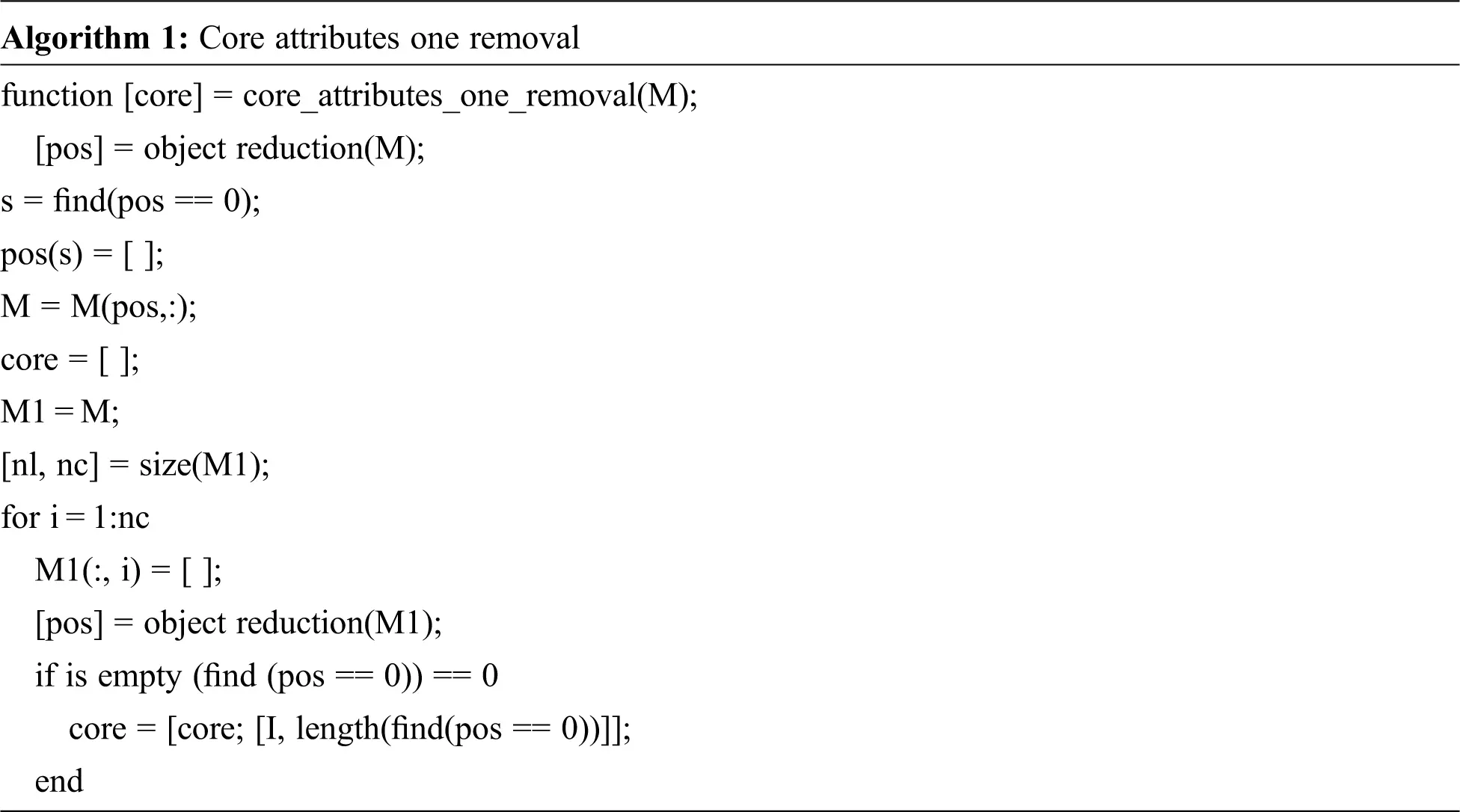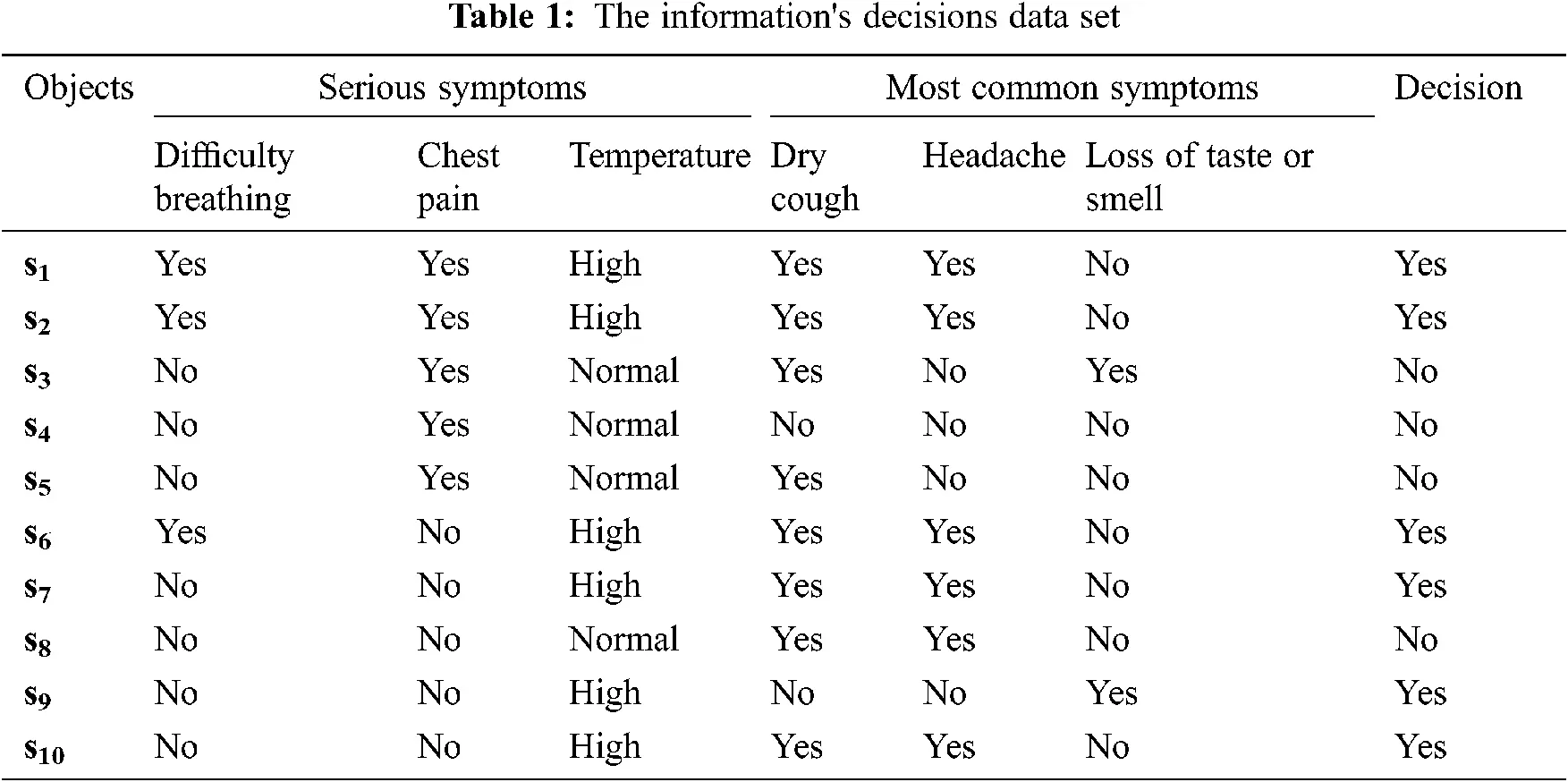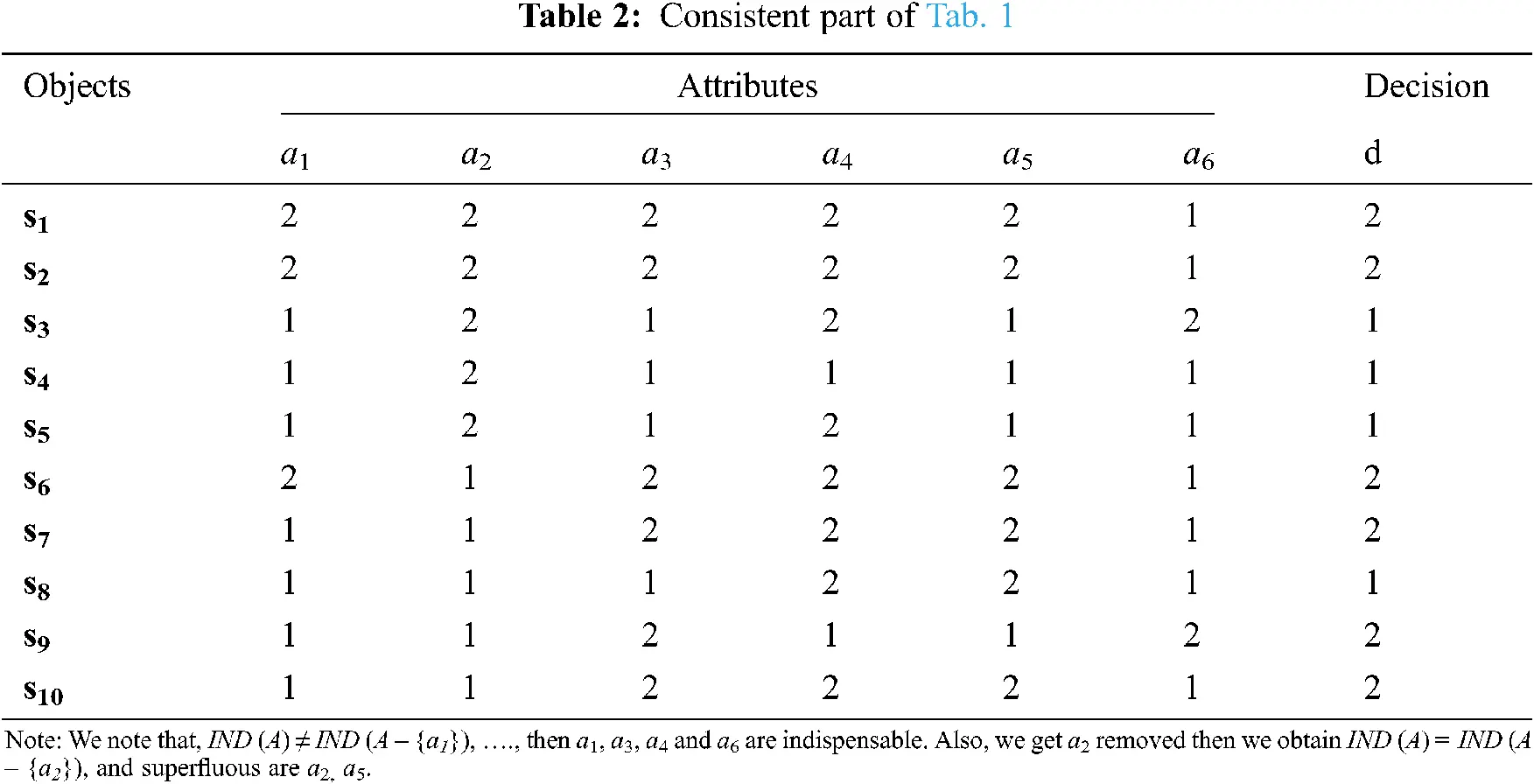Then, we get the removal of attributes as the next Tab. 3,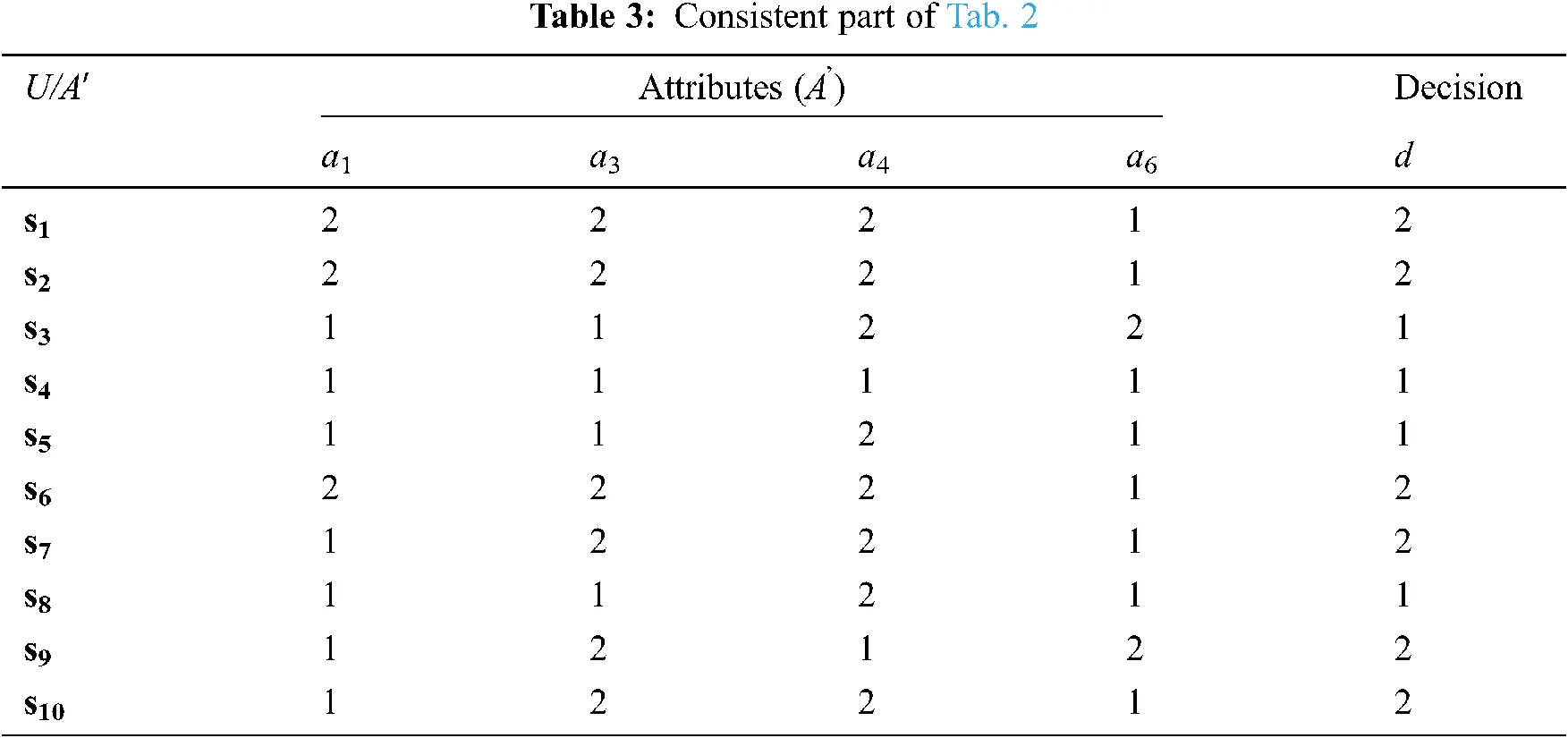From Tab. 3 we obtain the symptoms of every patient as follows:

V(s1) = {a1, a3, a4}, V(s2) = {a1, a3, a4}, V(s3) = {a4, a6}, V(s4) = ϕ , and V(s5) = {a4}. Now, we can generate the following relation: siRsj ⇔ V(si) ⊆ V(sj).

We apply this relation for all features in the table to induce the neighborhoods as follows.

R = {(s1, s1), (s1, s2), (s2, s2), (s2, s1), (s3, s3), (s4, s4), (s4, s1), (s4, s2), (s4, s3), (s4, s5), (s5, s5), (s5, s1), (s5, s2)}. Thus, the right neighborhoods of each element in U of this relation are Rr s1 = {s1, s2}, Rr s2 = {s1, s2},

Rr s3 = {s3}, Rr s4 =U, Rr s5 = { s1, s2, s3, s5}. Then the topology deduced from this relation is T = {U,  ,  {s1,  s2},  {s3},  {s1,  s2. s3},  {s1,  s2,  s3,  s5}}, Tc = {U,  ,  {s4,  s5},  {s4},  {s5,  s4. s3},  {s1,  s2,  s4,  s5}}.

Then, we construct the following Tab. 4 to obtain the class of simply* alpha open set,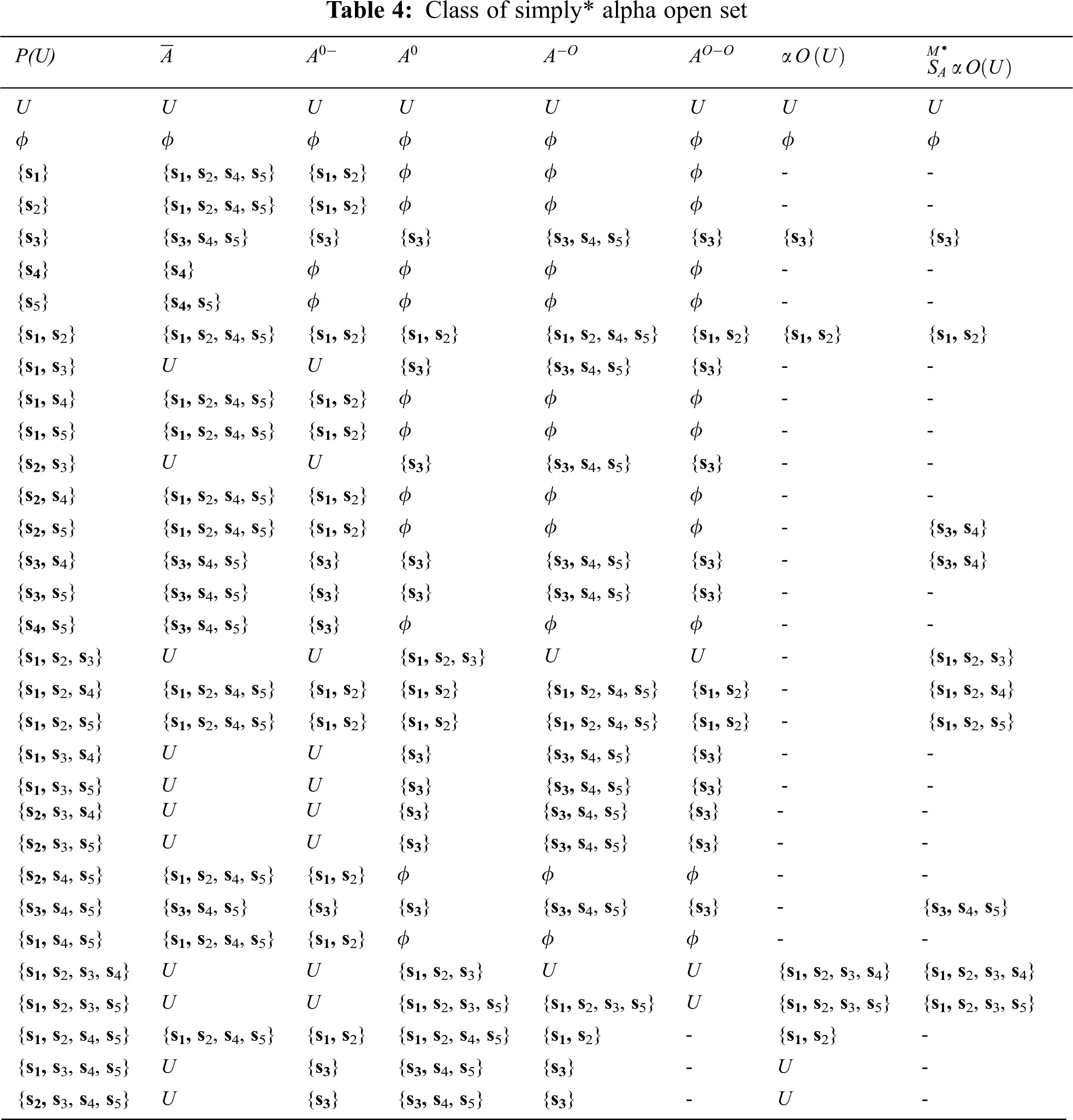Then the class of simply* alpha open set is SMαO(U)={U,ϕ,{s3},{s3,s5},{s1,s2,s3},{s1,s2,s4},{s1,s2,s5}}

Step 1: When the feature a1-Difficulty breathing is removed, the symptoms of every patient are: V(s1) = {a3,  a4},  V(s2) = {a3,  a4},  V(s3) = {a4,  a6},  V(s4) = , and V(s5) = {a4}.

Thus, the right neighborhoods of each element in U of this relation are.

Rr(s1)={s1,s2},Rr(s2)={s1,s2},Rr(s3)={s3},Rr(s4)=U,andRr(s5)={s1,s2,s3,s5}.

Then the topology deduced from this relation is T= {U,ϕ,{s1,s2},{s3},{s1,s2,s3},{s1,s2,s3,s5}} , Tc = {U,  ,  {s3,  s4,  s5},  {s1,  s2,  s4, s5},  {s4,  s5},  {s4} },  and hence the class of simply* alpha open set of the whole is identical with the class of simply* alpha open set without the symptom a1; this means that SAMαO(U)=SA{a1}MαO(U) .

Step 2: When the feature a3-Temperature is removed, the symptoms of every patient are: V(s1) = {a1,  a4},  V(s2) = {a1,  a4},  V(s3) = {a1,  a4,  a6},  V(s4) = {a1}, and V(s5) = {a1,  a4}.

Thus, the right neighborhoods of each element in U of this relation are.

Rr(s1) = {s1,  s2,  s3},  Rr(s2) = {s1,  s2,  s3},  Rr(s3) = {s3},  Rr(s4) = {s1,  s2,  s3,  s4},  and Rr(s5) = {s1,  s2,  s3,  s5}. Then, the topology deduced from this relation is T = {U,  ,  {s1,  s2,  s3},  {s1,  s2,  s3,  s4},  {s1,  s2,  s3,  s5}},  Tc = {U,  ,  {s4,  s5},  {s5},  {s4} },  and hence the class of simply* alpha open set of the whole is not identical with the class of simply* alpha open set without the symptom a3, if SAMαO(U)SA{a3}MαO(U) , as in Tab. 5,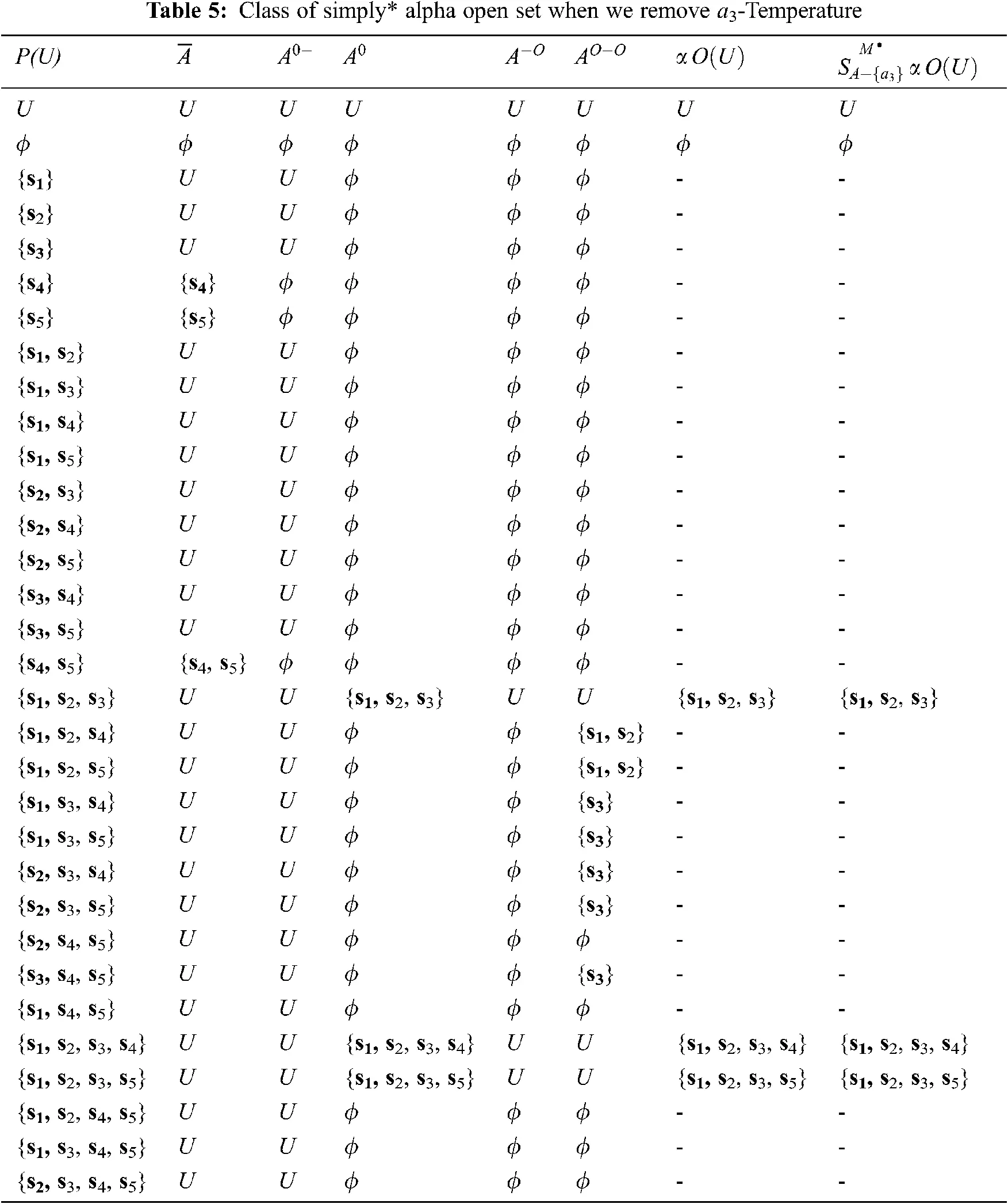Step 3: When the feature a4-Dry cough is removed, the symptoms of every patient are:

V(s1)={a1,a3},V(s2)={a1,a3},V(s3)={a1,a6},V(s4)={a1},andV(s5)={a1}.

Thus, the right neighborhoods of each element in U of this relation are Rr(s1) = {s1,  s2},  Rr(s2) = {s1,  s2},  Rr(s3) = {s3},  Rr(s4) = U,  and Rr(s5) = U.

Then the topology deduced from this relation is T = {U,  ,  {s1,  s2},  {s3},  {s1,  s2,  s3}},  Tc = {U,  ,  {s3,  s4,  s5},  {s1,  s2,  s4,  s5},  {s4,  s5}},  and hence the class of simply* alpha open set of the whole is identical with the class of simply* alpha open set without the symptom a4, this means that SAMαO(U)=SA{a4}MαO(U) . As the following Tab. 6,Step 3: When the feature a6-Loss of taste or smell is removed, the symptoms of every patient are: V(s1) = {a1,  a3},  V(s2) = {a1,  a3},  V(s3) = {a1,  a6},  V(s4) = {a1}, and V(s5) = {a1}.

Thus, the right neighborhoods of each element in U of this relation are Rr(s1) = {s1,  s2},  Rr(s2) = {s1,  s2},  Rr(s3) = {s3},  Rr(s4) = U,  and Rr(s5) = U.

Then the topology deduced from this relation is T = {U,  ,  {s1,  s2},  {s3},  {s1,  s2,  s3}},  Tc = {U,  ,  {s3,  s4,  s5},  {s1,  s2,  s4,  s5},  {s4,  s5}},  and hence the class of simply* alpha open set of the whole is not identical with the class of simply* alpha open set without the symptom a6-Loss of taste or smell this means that SAMαO(U)SA{a6}MαO(U) , as described in Tab. 7,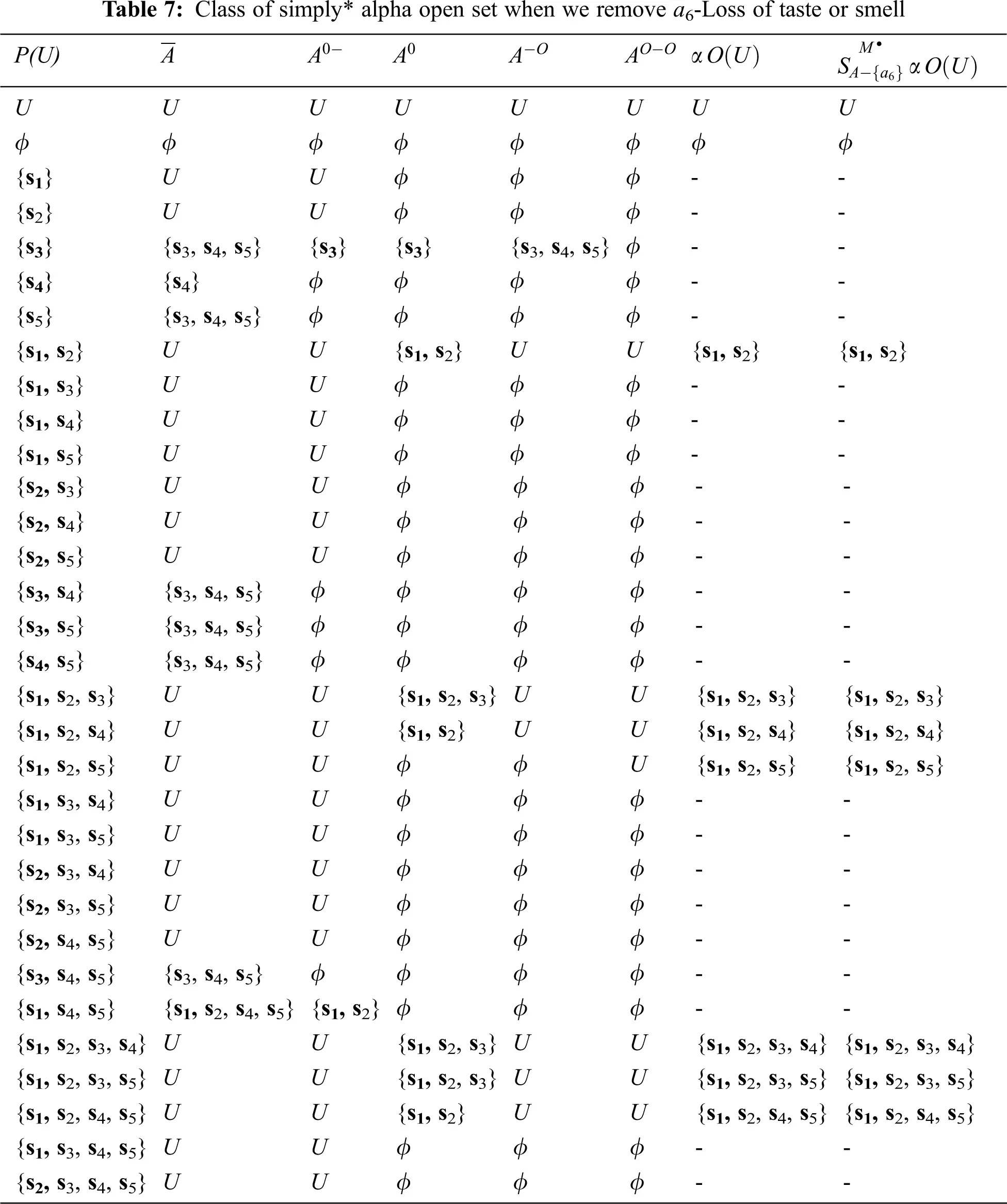Hence, from Steps (1–3), we observe that: the CORE is {a3,  a6}, that is the impact factors to determine Coronavirus infection are High Temperature and Loss of taste or smell.5  Conclusion

Our study in this article aims for defining a new type of simply* alpha generalized namely Simply*α -generalized closed, locally simply*α-generalized closed, and locally simply*α-generalized closed** sets. We show the relation between this type of set and the other of generalized open sets. We concluded that the generalized simple closed set contains most of the generalized closed sets and also, we defined new kinds of locally generalized simply closed sets based on simply* alpha open set. Furthermore, we studied the relation between these collections and other. We presented an application to a group of patients, where we presented a method using topological concepts to determine one of the main symptoms causing Coronavirus. Finally, using the class of simply alpha* open set, we identified the impact factors of Coronavirus infection and then we helped the physician to make an accurate decision making about patients. At the end of paper, this method has also proven effective in acquiring the basic symptoms that cause COVID-19. We plan to extend the proposed methodology in future work to include a multi-label classification issue.

Funding Statement: The authors extend their appreciation to the Deanship of Scientific Research at King Khalid University for funding this work through research groups program under Grant Number R.G.P. 1/206/42.

Conflicts of Interest: The authors declare that they have no conflicts of interest to report regarding the present study.

## References

1. G. Kampf, D. Todt, S. Pfaender and E. Steinmann, “Persistence of coronaviruses on inanimate surfaces and their inactivation with biocidal agents,” J. Hospital Infection, vol. 104, pp. 246–251, 2020.
2. S. A. Alblowi, M. El Sayed and M. A. El Safty, “Decision making based on fuzzy soft sets and its application in COVID-19,” Intelligent Automation & Soft Computing, vol. 30, no. 3, pp. 961–972, 2021.
3.    M. A. El Safty and S. AlZahrani, “Topological modeling for symptom reduction of coronavirus,” Punjab University Journal Mathematics, vol. 53, no. 3, pp. 47–59, 2021.
4.    L. X. Feng, S. L. Jing, S. K. Hu, D. F. Wang and H. F. Huo, “Modelling the effects of media coverage and quarantine on the COVID-19 infections in the UK,” Mathematical Biosciences and Engineering, vol. 17, no. 4, pp. 3618–3636, 2020.
5.    M. A. El Safty, M. El Sayed and S. A. Alblowi, “Accuracy based on simply* alpha open set in rough set and topological space,” Soft Computing, vol. 25, no. 16, pp. 10609–10615, 2021.
6.    M. A. El Safty, S. A. L. Zahrani, M. K. El-Bably and M. El Sayed, “Soft exai -rough set and its applications in decision making of coronavirus,” Computers, Materials & Continua, 2021.
7. M. El Sayed, M. A. El Safty and M. K. El-Bably, “Topological approach for decision-making of COVID-19 infection via a nano topology model,” AIMS Mathematics, vol. 6, no. 7, pp. 7872–7894, 2021.
8. M. A. El Safty, “Modeling uncertainty knowledge of the topological methods,” Poincare Journal of Analysis & Applications, vol. 8, no. 1, pp. 89–102, 2021.
9. M. A. Elsafty and A. M. Alkhathami, “A topological method for reduction of digital information uncertainty,” Soft Computing, vol. 24, no. 1, pp. 385–396, 2020.
10. M. E. Abd Monsef and R. A. Mahmoud, “On generalized continuous mappings,” Delta J. Science., vol. 8, no. 2, pp. 407–420, 1984.
11. T. N. Alharthi and M. A. El Safty, “Attribute topologies based similarity,” Cogent Mathematics, vol. 3, no. 1, pp. 1–11, 2016.
12. M. A. El Safty, A. A. Mousa, S. A. Alblowi and M. El Sayed, “Topological approach on fuzzy soft β-closure and β-interior and its application in decision making,” Nanoscience and Nanotechnology Letters, vol.12, no. 11, pp. 1323–1328, 2020.
13. M. A. El Safty, “Topological method for a study of discriminating three categories of banks and its use in attributes reduction,” International Journal of Scientific Research in Science, Engineering and Technology, vol. 7, no. 5, pp. 221–233, 2020.
14. K. Balachandran, P. Sundaram and H. Maki, “Generalized locally closed sets and GLC-continuous function,” Indian J. Pure & Applied Mathematics, vol. 27, no. 3, pp. 235–244, 1996.
15. M. Ganster and I. Reilly, “Locally closed sets and LC-continuous function,” International Journal of Mathematics and Mathematical Sciences, vol. 12, no. 3, pp. 417–424, 1989.
16. M. Ganster, I. Reilly and M. K. Vamanamurthy, “Remarks on locally closed sets,” Mathematics Pannonica, vol. 3, no. 2, pp. 107–113, 1992.
17. J. Cao, M. Ganster and I. Reilly, “On generalized closed sets,” Topology and its Applications, vol. 123, no. 1, pp. 37–46, 2002.This work is licensed under a Creative Commons Attribution 4.0 International License, which permits unrestricted use, distribution, and reproduction in any medium, provided the original work is properly cited.